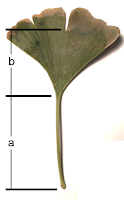## Saturday, October 20, 2012

### Golden Ratio in Nature

In words, the golden ratio is:
The ratio of the sum of two quantities over the larger quantity is equal to the ratio of the larger quantity over the smaller one.
The golden ratio expressed as an equation is:

(a + b) / a = 1.618 = a / b

From the golden ratio, we get a mathematical constant - a number. It is the number 1.6 which, by convention, is represented by the Greek letter φ (pronounced "fee").

The number φ is a mathematical curiosity. Like the mathematical constant π, φ appears everywhere.Ginkgo Leaf
The good, of course, is always beautiful, and the beautiful never lacks proportion.
Plato
The golden ratio is found in the proportions of leaves. I measured the golden ratio in the leaf of a ginkgo tree.

Using the typographical units of pica, I measured 28 pica from the notch to the base of the stem of a ginkgo leaf. I then measured 17 pica from the top of the stem to the base of the stem (length a).

The quotient of 28 pica over 17 pica yields the golden ratio 1.6.

Scientists have recently observed nanoscopic symmetry. The symmetry they observed had the attributes of the golden ratio, demonstrating this curious proportion at the quantum level.
The universe cannot be read until we have learnt the language and become familiar with the characters in which it is written. It is written in mathematical language, and the letters are triangles, circles and other geometrical figures, without which means it is humanly impossible to comprehend a single word.
Galileo Galilei
References